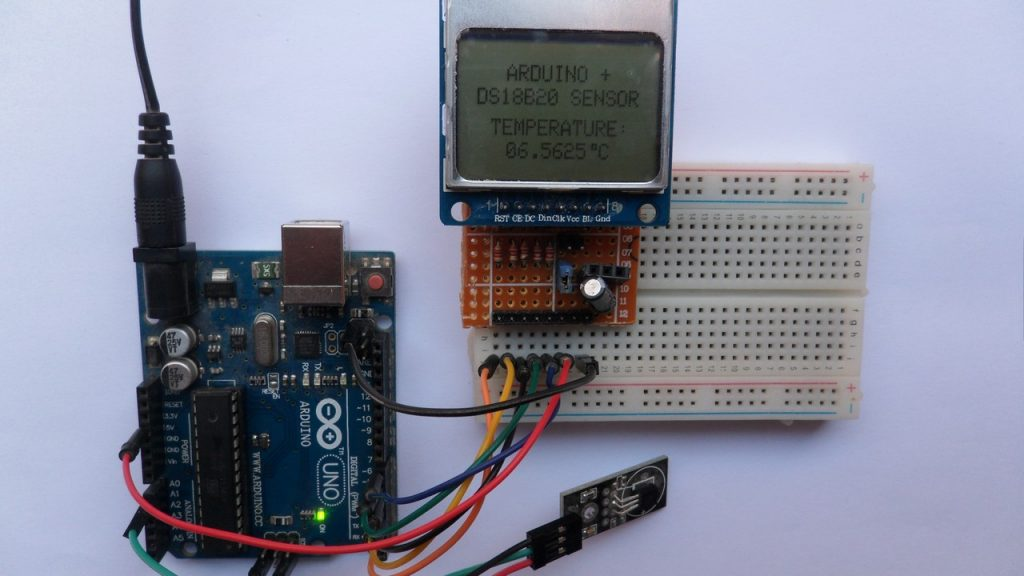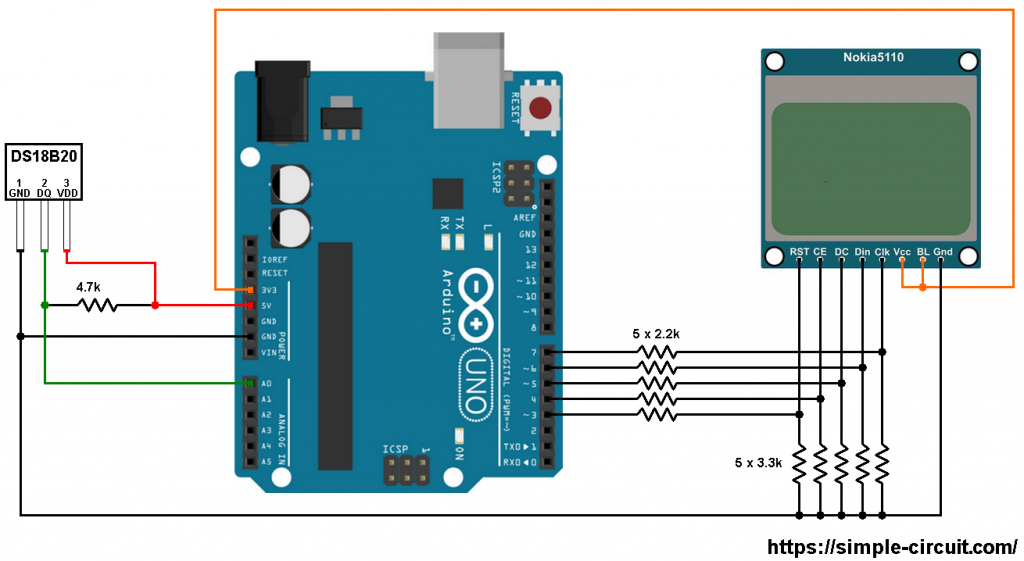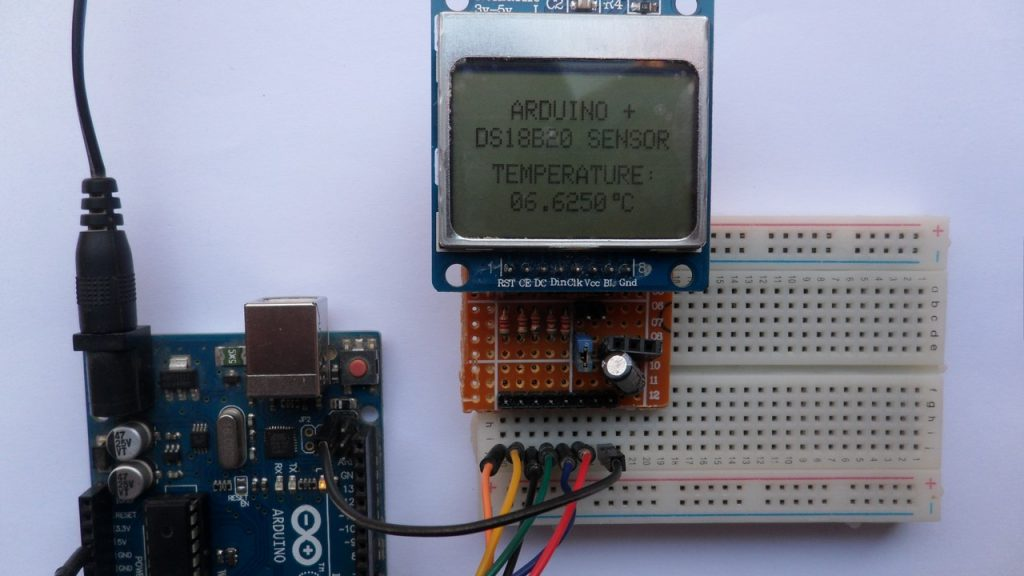# Arduino Thermometer with DS18B20 sensor and NOKIA 5110 LCD

This Arduino tutorial shows how to build a temperature measurement station using DS18B20 sensor and NOKIA 5110 (NOKIA 3310) LCD screen.

The DS18B20 temperature sensor is a 3-pin electronic component (like a simple transistor) from Maxim (formerly Dallas) which uses 1-wire protocol to communicate with master device (microprocessor, microcontroller ….). Each DS18B20 device has a unique 64-bit serial code, which allows multiple DS18B20s to function on the same 1-wire bus and controlled with one master device.
The DS18B20 sensor provides 9-bit to 12-bit Celsius temperature measurement resolution (programmable resolution).

To see how to interface Arduino with Nokia 5110 LCD, visit the following post:
Interfacing Arduino with Nokia 5110 LCD

And to see how to interface Arduino with DS18B20 sensor for the first time, take a look at this post:
Digital thermometer using Arduino and DS18B20 sensor

Hardware Required:

• Arduino board
• Nokia 5110 LCD screen
• DS18B20 temperature sensor   —->   datasheet
• 5 x 3.3k ohm resistor
• 5 x 2.2k ohm resistor
• 4.7k ohm resistor
• Jumper wiresArduino with DS18B20 sensor and Nokia 5110 LCD circuit:
The following image shows project circuit schematic diagram.

The DS18B20 sensor has 3 pins: VCC, data and GND where:
VCC: sensor power supply pin, connected to Arduino 5V pin,
data pin: connected to Arduino analog pin 0 (A0) and
GND: connected to Arduino GND pin.

A pull-up resistor of 4.7k ohm is required because the DS18B20 output is open drain.The Nokia 5110 LCD which is shown in the circuit diagram has 8 pins (from left to right): RST (reset), CE (chip enable), DC (or D/C: data/command), Din (data in), Clk (clock), VCC (3.3V), BL (back light) and Gnd (ground).

This LCD works with 3.3V only (power supply and control lines). The LCD module is supplied with 3.3V which comes from the Arduino board (VCC pin of the LCD is connected to Arduino 3.3V pin), BL pin is also connected to 3.3V.

All Arduino UNO board output pins are 5V, connecting a 5V pin to the Nokia 5110 LCD could damage its controller circuit.
To connect the Arduino to the LCD module and for the LCD safety, I used voltage divider for each line which means there are 5 voltage dividers. Each voltage divider consists of 2.2k and 3.3k resistors, this drops the 5V into 3V which is sufficient.

Nokia 5110 LCD pins are connected to Arduino UNO board as follows (each one through voltage divider):
RST (reset) pin is connected to Arduino digital pin 3
CE (chip enable) pin is connected to Arduino digital pin 4
DC (data/command) pin is connected to Arduino digital pin 5
DIN (data in)  pin is connected to Arduino digital pin 6
CLK (clock) pin is connected to Arduino digital pin 7
VCC and BL are connected to Arduino 3V3 pin
GND is connected to Arduino GND pin.

Arduino with DS18B20 sensor and Nokia 5110 LCD code:
The Arduino code below doesn’t use any library for the DS18B20 sensor.

The following Arduino code requires 2 libraries from Adafruit Industries:
The first library is a driver for the Nokia 5110 LCD (PCD8544 controller) which can be installed from Arduino IDE library manager (Sketch —> Include Library —> Manage Libraries …, in the search box write “nokia” and install the one from Adafruit).

The second library is Adafruit graphics library which can be installed also from Arduino IDE library manager.

The same thing for the other library file.

In the code there are total of 3 libraries, they’re included in the code as follows:

Nokia 5110 LCD connection with the Arduino is configured as shown below:

and the DS18B20 sensor data pin is defined as:

Functions used in the code:
bool ds18b20_start(): used to know if the DS18B20 sensor is correctly connected to the circuit, returns 1 if OK and 0 if error.
ds18b20_write_bit(bool value): writes (sends) 1 bit to the DS18B20 sensor, the bit is ‘value’ which may be 1 or 0.
ds18b20_write_byte(byte value): writes 1 byte (8 bits) to the DS18B20 sensor, this function is based on the previous function. This function writes LSB first.
byte ds18b20_read_byte(void): reads 1 byte from the DS18B20 sensor, this function is based on the previous function. This function reads LSB first.
bool ds18b20_read(int *raw_temp_value): reads the temperature raw data which is 16-bit long (two 8-bit registers), the data is stored in the variable raw_temp_value, returns 1 if OK and 0 if error.

The value of the temperature in degree Celsius is equal to the raw value divided by 16 (in case of 12-bit resolution). The default resolution of the DS18B20 is 12 bits.

Full Arduino code:

The following image shows the result of my protoboard circuit:The video below shows Proteus simulation (note that simulation circuit is not the same as real hardware circuit, hardware circuit diagram is shown above):